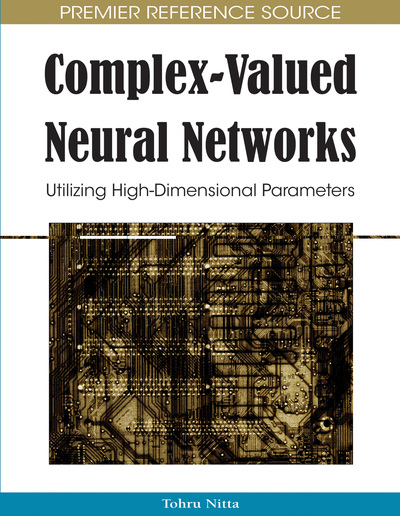# Quaternionic Neural Networks: Fundamental Properties and Applications

Teijiro Isokawa (University of Hyogo, Japan), Nobuyuki Matsui (University of Hyogo, Japan) and Haruhiko Nishimura (University of Hyogo, Japan)
DOI: 10.4018/978-1-60566-214-5.ch016

## Abstract

Quaternions are a class of hypercomplex number systems, a four-dimensional extension of imaginary numbers, which are extensively used in various fields such as modern physics and computer graphics. Although the number of applications of neural networks employing quaternions is comparatively less than that of complex-valued neural networks, it has been increasing recently. In this chapter, the authors describe two types of quaternionic neural network models. One type is a multilayer perceptron based on 3D geometrical affine transformations by quaternions. The operations that can be performed in this network are translation, dilatation, and spatial rotation in three-dimensional space. Several examples are provided in order to demonstrate the utility of this network. The other type is a Hopfield-type recurrent network whose parameters are directly encoded into quaternions. The stability of this network is demonstrated by proving that the energy decreases monotonically with respect to the change in neuron states. The fundamental properties of this network are presented through the network with three neurons.
Chapter Preview
Top

## Background

Several systems for hypercomplex numbers have been investigated, such as quaternion, octonion, sedenion. They can be described as special cases of Clifford algebra (Porteous, 1995) (Lounesto, 2001). Quaternions are hypercomplex numbers of rank four; they are the four-dimensional extensions of imaginary numbers discovered by Sir William Rowan Hamilton (Graves, 1975) (Hankins, 1980) (Bell, 1999), which have been extensively used in modern mathematics, signal processing, computer graphics, robotics, etc (Lambek, 1995) (Mukundan, 2002) (Hoggar, 1992) (Kuipers, 1998) (Previn & Webb, 1983) (Sahul, Biswall & Subudhi, 2008) (Bülow & Sommer, 2001). Quaternion is also defined as a special case of Clifford algebra, but the representation of quaternion is simple and easy to understand its geometrical meanings. It has been found that 3D geometrical affine transformations—translation, dilatation, and spatial rotation—in three-dimensional space can be represented compactly and efficiently by the operators of quaternions. In the following section, we recapitulate the basic definitions, operations, and notations of quaternions. The 3D geometrical affine transformation realized by quaternions, which is used in the multilayer perceptron model in this chapter, is also described. For the detailed properties and applications of quaternions, please refer to the literatures (Lambek, 1995) (Mukundan, 2002) (Hoggar, 1992) (Kuipers, 1998).

## Complete Chapter List

Search this Book:
Reset